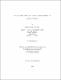## Wolff's ideal problem in the multiplier algebra on dirichlet space2014
##### Publisher
University of Alabama Libraries
##### Abstract

In an effort to classify ideal membership for finitely-generated ideals in H&infin (D), Wolff [G] proved that: If {f j}j=1 j=n&subset H&infin (D), H &belongs H&infin (D) and |H(z)| &le (&sum |fj}(z)| 2)&half for all z in D then H 3&belongs I ({fj}), the ideal generated by {fj} in H&infin (D). In the first part of this dissertation, we establish an analogue of Wolff's result for the algebra of multipliers on Dirichlet space. In the second part, we extend this result to the multiplier algebra on weighted Dirichlet space with the weight &alpha in (0,1). Finally, we will prove a general ideal problem. We provide the best known sufficient condition for when H itself is in the ideal.

##### Description
Electronic Thesis or Dissertation
Mathematics